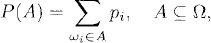# EVENTS AND RANDOM VARIABLES

Samples, events, and random variables are the fundamental concepts in probability theory. In this chapter, we shall introduce the definitions of these concepts based on measure theory.

# 11.1 Basic Concepts and Facts

Definition 11.1 (Sample Space, Sample Point, and Event). Let (Ω,, P) be a probability space (see Definition 2.12). The set Ω is called the sample space, a point in Ω is called a sample point, and a subset ofis called an event.

Definition 11.2 (Discrete Probability Space). Let Ω = {ω1, ω2,…} be a finite or countably infinite set. Let P be a set function defined asandwhere p1, p2,… are nonnegative numbers whose sum is 1. Then P is a probability measure on 2Ω, which is the collection of all subsets of Ω. The probability space (Ω, 2Ω, P) is called a discrete probability space.

Definition 11.3 (Almost Surely). Letbe a probability space. A statement about outcomes is said to be true almost surely (a.s.), or with probability 1, if the set ...

Get Measure, Probability, and Mathematical Finance: A Problem-Oriented Approach now with the O’Reilly learning platform.

O’Reilly members experience live online training, plus books, videos, and digital content from nearly 200 publishers.